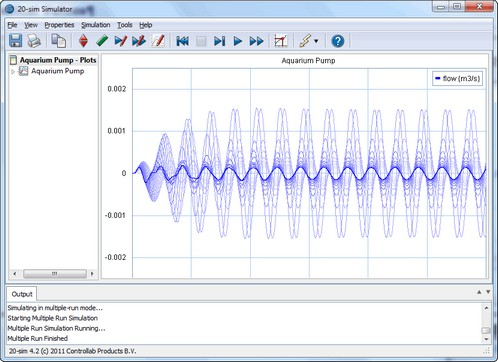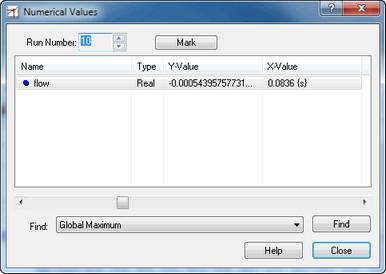﻿ 20-sim webhelp > Simulator > Running a Simulation > Numerical Values

# Numerical Values

In every simulation plot you can inspect the numerical values by selecting the Numerical Values command of the View menu. The Numerical Values window, can also be popped up by double clicking the left mouse button, while pointing the mouse at a curve in the plot.The Numerical Values window will pop up and display the numerical values of all plotted variables as a function of time. The last variable shown is always the X-axis variable (usually time).The Numerical Values window.

 • Run Number: The run number indicates the run of which the numerical values are shown. The highest number indicates the last run simulated. Lower numbers are used for previous runs. To select another run number, type in the number of interest or point in the plot at the curve of interest. In the simulation plot, previous runs are shown with fading colors.
 • List: The list shows all variables that are shown in the plot and their corresponding X-values and Y-values. The variable that is currently selected is pointed out by an arrow in the plot.
 • Scroll-bar: Use the scroll-bar to change the selected x-axis variable value (usually time).
 • Find: With the Find button, the global maximum etc. of the selected variable can be found. The following functions can be selected:
 • Global Maximum
 • Global Minimum
 • Next Local Maximum
 • Next Local Minimum
 • Previous Local Maximum
 • Previous Local Minimum
 • Mark: Click the Mark button to store he set of numerical values. If you drag the scroll-bar to find a new set of values, the relative changes will be displayed as well as a first order derivative.